## Sharing Problem

A problem also known as the Points Problem or Unfinished Game. Consider a tournament involving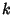players playing the same game repetitively. Each game has a single winner, and denote the number of games won by playerat some juncture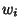. The games are independent, and the probability of theth player winning a game is. The tournament is specified to continue until one player has wongames. If the tournament is discontinued before any player has wongames so that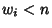for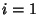, ...,, how should the prize money be shared in order to distribute it proportionally to the players' chances of winning?

For player, call the number of games left to winthe quota.'' For two players, let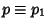and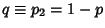be the probabilities of winning a single game, andandbe the number of games needed for each player to win the tournament. Then the stakes should be divided in the ratio, where(1)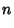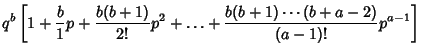(2)

(Kraitchik 1942).

Ifplayers have equal probability of winning (cell probability''), then the chance of playerwinning for quotas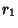, ...,is(3)

where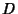is the Dirichlet Integral of type 2D. Similarly, the chance of playerlosing is(4)

whereis the Dirichlet Integral of type 2C. If the cell quotas are not equal, the general Dirichlet integralmust be used, where(5)

Ifand, thenand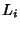reduce toas they must. Let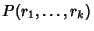be the joint probability that the players would be Ranked in the order of thes in the argument list if the contest were completed. For,(6)

Forwith quota vectorand,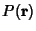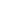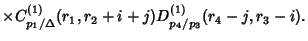(7)

An expression foris given by Sobel and Frankowski (1994, p. 838).

Kraitchik, M. The Unfinished Game.'' §6.1 in Mathematical Recreations. New York: W. W. Norton, pp. 117-118, 1942.
Sobel, M. and Frankowski, K. The 500th Anniversary of the Sharing Problem (The Oldest Problem in the Theory of Probability).'' Amer. Math. Monthly 101, 833-847, 1994.